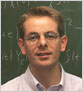Mathematical Institutepublications

seminar: Evolution equations in probability spacespre-Class Mathematics starts 24 January 2017, 3 p.m. in Snellius 403.

Since January 2006 I am an assistant professor ("universitair docent") at the Mathematical Institute of Leiden University. I have been supported by a Vidi-grant from the Netherlands Organisation for Scientific Research (NWO). The topic is "Stationary dynamics in infinite dimensions".

My research interests are

• functional analysis: partially ordered vector spaces, isometric theory of Banach spaces
• stochastic differential equations (SDE): invariant measures, SDE with delay, semimartingales, stochastic integration in Banach spaces
and any other topic catching my attention. For instance, nonexpansive dynamical systems and measure-valued evolution equations.

• Dr. O.W. van Gaans
Mathematical Institute
Leiden University
P.O. Box 9512
2300 RA Leiden
The Netherlands
• Phone: +31 71 5277122
Fax: +31 71 5277101
• Email: